Tamilnadu State Board New Syllabus Samacheer Kalvi 11th Physics Guide Pdf Chapter 7 Properties of Matter Text Book Back Questions and Answers, Notes.

## Tamilnadu Samacheer Kalvi 11th Physics Solutions Chapter 7 Properties of Matter

### 11th Physics Guide Properties of Matter Book Back Questions and AnswersI. Multiple choice questions:

Question 1.
Consider two wires X and Y. The radius of wire X is 3 times the radius of Y. If they are stretched by the same load then the stress on Y is:
(a) equal to that on X
(b) thrice that on X
(c) nine times that on X
(d) Half that on X
(c) nine times that on X

Hint:Question 2.
If a wire is stretched to double of its original length, then the strain in the wire is:
(a) 1
(b) 2
(c) 3
(d) 4
(a) 1

Hint:Question 3.
The load – elongation graph of three wires of the same material are shown in figure. Which of the following wire is the thickest?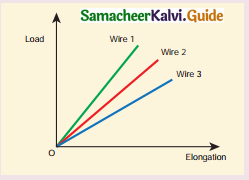(a) wire 1
(b) wire 2
(c) wire 3
(d) all of them have same thickness
(a) wire 1

Hint:
For wire stress is less
Stress = $$\frac { Force }{ Area }$$
As stress is less Area will be greater.
∴ Wire 1 is thickest.Question 4.
For a given material, the rigidity modulus is [ $$\frac { 1 }{ 3 }$$ ]3 of Young’s modulus. Its Poisson’s ratio:
(a) 0
(b) 0.25
(c) 0.3
(d) 0.5
(d) 0.5

Hint: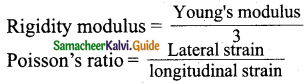Question 5.
A small sphere of radius 2 cm falls from rest in a viscous liquid. Heat is produced due to viscous force. The rate of production of heat when the sphere attains its terminal velocity is proportional to: [NEET model 2018]
(a) 2²
(b) 2³
(c) 24
(d) 25
(d) 25

Hint:Question 6.
Two wires are made of the same material and have the same volume. The area of cross sections of the first and the second wires are A and 2A respectively. If the length of the first wire is increased by Δl on applying a force F, how much force is needed to stretch the second wire by the same amount? [NEET model 2018]
(a) 2
(b) 4
(c) 8
(d) 16
(b) 4

Question 7.
With an increase in temperature, the viscosity of liquid and gas, respectively will:
(a) increase and increase
(b) increase and decrease
(c) decrease and increase
(d) decrease and decrease
(c) decrease and increaseQuestion 8.
The Young’s modulus for a perfect rigid body is:
(a) 0
(b) 1
(c) 0.5
(d) infinity
(d) infinity

Question 9.
Which of the following is not a scalar?
(a) viscosity
(b) surface tension
(c) pressure
(d) stress
(d) stress

Question 10.
If the temperature of the wire is increased, then the Young’s modulus will:
(a) remain the same
(b) decrease
(c) increase rapidly
(d) increase by very a small amount
(b) decrease

Question 11.
Copper of fixed volume V is drawn into wire of length l. When this wire is subjected to a constant force F, the extension produce in the wire is ∆l. If Y represents the Young’ modulus, then which of the following graph is a straight line?
(a) ∆l verses V
(b) ∆l verses Y
(c) ∆l verses F
(d) ∆l verses $$\frac { 1 }{ l }$$
(c) ∆l verses F

Hint:
Strain ∝ Stress
∴ ∆l ∝ FQuestion 12.
A certain number of spherical drops of a liquid of radius R coalesce to form a single drop of radius R and volume V If T is the surface tension of the liquid, then:
(a) energy = 4VT($$\frac { 1 }{ r }$$ – $$\frac { 1 }{ R }$$) is released
(b) energy = 3 VT($$\frac { 1 }{ r }$$ + $$\frac { 1 }{ R }$$) is absorbed
(c) energy = 3VT ($$\frac { 1 }{ r }$$ – $$\frac { 1 }{ R }$$)is released
(d) energy is neither released nor absorbed
(c) energy = 3VT ($$\frac { 1 }{ r }$$ – $$\frac { 1 }{ R }$$)is released

Question 13.
The following four wires are made of the same material. Which of these will have the largest extension when the same tension is applied?
(a) length = 200 cm, diameter = 0.5 mm
(b) length= 200 cm, diameter = 1 mm
(c) length 200 cm, diameter = 2 mm
(d) length= 200 cm, diameter = 3 m
(a) length = 200 cm, diameter = 0.5 mm

Hint:Hence the value of l must be most and that of d must be least.

Question 14.
The wettability of a surface by a liquid depends primarily on:
(a) viscosity
(b) surface tension
(c) density
(d) angle of contact between the surface and the liquid
(d) angle of contact between the surface and the liquid

Question 15.
In a horizontal pipe of non-uniform cross section, water flows with a velocity of 1 ms-1 at a point where the diameter of the pipe is 20 cm. The velocity of water (ms-1) at a point where the diameter of the pipe 10 cm is:
(a) .0025 m/s
(b) .25 m/s
(c) 0.025 m
(d) .5 m/s
(b) .25 m/s

Hint:Question 1.
Define stress and strain.
Stress: The force per unit area is called as stress.
Stress, σ = $$\frac { Force }{ Area }$$ = $$\frac { F }{ A }$$

Strain: Strain is defined as the ratio of change in size to the original size of an object. It measures the degree of deformation.Question 2.
State Hooke’s law of elasticity.
It states that for small deformation, the stress is directly proportional to strain.Question 3.
Define Poisson’s ratio.
It is defined as the ratio of relative contraction (lateral strain) , to relative expansion (longitudinal strain).Question 4.
Explain elasticity using intermolecular forces.
Elastic behaviour of solid. In a solid, atoms and molecules are arranged in such a way that each molecule is acted upon by the forces due to the neighbouring molecules. When deforming force is applied on a body so that its length increases, then the molecules of the body go far apart.

Question 5.
Which one of these is more elastic, steel or rubber? Why?
Steel is more elastic than rubber. If equal stress is applied to both steel and rubber, the steel produces less strain. So Young’s modulus is higher for steel than rubber. Hence steel is more elastic than rubber.

Question 6.
A spring balance shows wrong readings after using for a long time. Why?
When a spring balance has been used for a long time, it develops elastic fatigue, the spring of such a balance takes a longer time to recover its original configuration and therefore it does not give correct measurement.Question 7.
What is the effect of temperature on elasticity?
As the temperature of the substance increases, its elasticity decreases.

Question 8.
Write down the expression for the elastic potential energy of a stretched wire.
W = $$\frac { 1 }{ 2 }$$ Fl = Elastic potential energy

Question 9.
State Pascal’s law in fluids.
If the pressure in a liquid is changed at a particular point, the change is transmitted to the entire liquid without being diminished in magnitude.

Question 10.
State Archimedes principle.
When a body is partially or wholly immersed in a fluid, it experiences an upward thrust equal to the weight of the fluid displaced by it and its upthrust acts through the centre of gravity of the liquid displaced.
Upthrust or buoyant force = weight of liquid displaced.

Question 11.
What do you mean by upthrust or buoyancy?
The upward force exerted by a fluid that opposes the weight of an immersed object in a fluid is called upthrust or buoyant force.Question 12.
State the law of floatation.
The law of floatation states that a body will float in a liquid if the weight of the liquid displaced by the immersed part of the body equals the weight of the body.

Question 13.
Define coefficient of viscosity of a liquid.
The coefficient of viscosity of a liquid is defined as the viscous force acting tangentially per unit area of a liquid layer having a unit velocity gradient in a direction perpendicular to the direction of flow of the liquid.

Question 14.
Distinguish between streamlined flow and turbulent flow.
Streamlined flow:

• When a liquid flows such that each particle of the liquid passing a point moves along the same path and has the same velocity as its predecessor then flow is said to be streamlined flow.
• The velocity of the particles is constant.
• The path taken by the particle in this flow is a curve.

Turbulent flow:

• When the speed of the moving liquid, exceeds the critical speed vc, the motion becomes turbulent.
• The velocity changes both in magnitude and direction from particle to particle.
• The path taken by the particle in this flow becomes erratic and whirlpool. Like circles.

Question 15.
What is Reynold’s number? Give its significance.
Reynold’s number Rc is a critical variable, which decides whether the flow of a fluid through a cylindrical pipe is streamlined or turbulent.
Rc = $$\frac { ρVD }{ η }$$Question 16.
Define terminal velocity.
The maximum constant velocity acquired by a body while falling freely through a viscous medium is called the terminal velocity VT.

Question 17.
Write down the expression for Stoke’s force and explain the symbols involved in it.
F= 6πηrv

• Radius (r ) of the sphere
• Velocity (v) of the sphere and
• Coefficient of viscosity η of the liquid.

Question 18.
State Bernoulli’s theorem.
According to Bernoulli’s theorem, the sum of’ pressure energy, kinetic energy, and potential energy per unit mass of an incompressible. Non-viscous fluid in a streamlined flow remains a constant.

Question 19.
What are the energies possessed by a liquid? Write down their equations.
A liquid in a steady flow can possess three kinds of energy. They are
(i) Kinetic energy, KE = $$\frac { 1 }{ 2 }$$mv²
(ii) Potential energy, PE = mgh
(iii) Pressure energy, respectively Ep = PV

Question 20.
Two streamlines cannot cross each other. Why?
If two streamlines cross each other, there will be two directions of flow at the point of intersection which is impossible.Question 21.
Define surface tension of a liquid. Mention its S.l unit and dimension.
Surface tension is defined as the force acting on a unit length of an imaginary line drawn on the free surface of the liquid, the direction of the force being perpendicular to the line so drawn and acting parallel to the surface. The SI unit and dimensions of surface tension are Nm-1 and MT-2, respectively.

Question 22.
How is surface tension related to surface energy?
The surface energy per unit area of a surface is numerically equal to the surface tension.

Question 23.
Define the angle of contact for a given pair of solid and liquid.
The angle between tangents drawn at the point of contact to the liquid surface and solid surface inside the liquid is called the angle of contact for a pair of solid and liquid. It is denoted by θ.

Question 24.
Distinguish between cohesive and adhesive forces.
Cohesive – The force between the like molecules which holds the liquid together is called ‘cohesive force’.

Adhesive – When the liquid is in contact with a solid, the molecules of these solid and liquid will experience an attractive force which is called ‘adhesive force’.

Question 25.
What are the factors affecting the surface tension of a liquid?

1. The presence of any contamination or impurities.
2. The presence of dissolved substances.
3. Electrification
4. TemperatureQuestion 26.
What happens to the pressure inside a soap bubble when air is blown into it?
Pressure will slowly increase inside the soap bubble.

Question 27.
What do you mean by capillarity or capillary action?
In a liquid whose angle of contact with solid is less than 90°, suffers capillary rise. On the other hand, in a liquid whose angle of contact is greater than 90°, suffers capillary fall. The rise or fall of a liquid in a narrow tube is called capillarity or capillary action.

Question 28.
A drop of oil placed on the surface of water spreads out. But a drop of water place on oil contracts to a spherical shape. Why?
When a drop of water is placed on oil, the cohesive force of water molecules dominates the adhesive force between water and oil molecules. Hence drop of water contracts to a spherical shape.

Question 29.
State the principle and usage of Venturimeter.
This device is used to measure the rate of flow (or say flow speed) of the incompressible fluid flowing through a pipe. It works on the principle of Bernoulli’s theorem.Question 1.
State Hooke’s law and verify it with the help of an experiment.
Hooke’s law states that for a small deformation when the stress and strain are proportional to each other.

It can be verified in a simple way by stretching a thin straight wire (stretches like spring) of length L and uniform cross-sectional area A spring is suspended from a fixed point O. A pan and a pointer are attached at the free end of the wire as shown in Figure.

Using a vernier scale arrangement the extension produced on the wire is measured. From the experiment, it is known that for a given load, the corresponding stretching force is F and the elongation produced on the wire is ΔL.It is directly proportional to the original length L and inversely proportional to the area of cross-section A. A graph is plotted using F on the X-axis and AL on the Y-axis. This graph is a straight line passing through the origin as shown in Figure.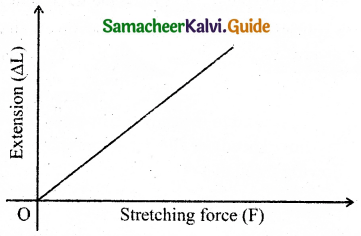Variation of ∆L with F
∴ ∆L = (slope)F
Multiplying and dividing by volume,
V = AL,
F(slope) = $$\frac { AL }{ AL }$$ ∆L
Rearranging, we geti.e., the stress is proportional to the strain in the elastic limit.

Question 2.
Explain the different types of modulus of elasticity.
There are three types of modulus of elasticity. They are:

1. Young’s modulus
2. Bulk modulus
3. Rigidity modulus

Young’s modulus: When a wire is stretched or compressed, then the ratio between tensile stress (or compressive stress) and tensile strain (or compressive strain) is defined as Young’s modulus.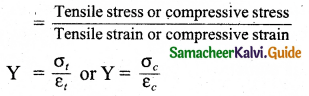SI unit of Young’s modulus is Nm-2 or pascal.
Bulk modulus: Bulk modulus is defined as the ratio of volume stress to the volume strain.
Bulk modulus,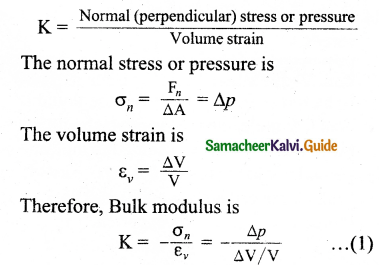The negative sign in equation (1) means that when pressure is applied to the body, its volume decreases.
The rigidity modulus or shear modulus:
It is defined as rigidity modulus or Shear modulus,Question 3.
Derive an expression for the elastic energy stored per unit volume of a wire.
When a body is stretched, work is done against the restoring force (internal force). This work done is stored in the body in the form of elastic energy. Let us consider a wire whose un-stretch length is L and the area of cross-section is A. Let a force produce an extension l and it is assumed that the elastic limit of the wire has not been exceeded and there is no loss in energy. Then, the work done by the force F is equal to the energy gained by the wire. The work is done in stretching the wire by dl,
dW = F dl
The total work done in stretching the wire from 0 to l is
W = $$\int_{0}^{l} \mathrm{~F} d l$$ … (1)
From Young’s modulus of elasticity,
Y = $$\frac { F }{ A }$$ x $$\frac { L }{ l }$$ ⇒ F = $$\frac { YAl }{ L }$$ … (2)
Substituting equation (2) in equation (1), we get
W = $$\int_{0}^{l} \frac{\mathrm{YAl}}{\mathrm{L}} d l$$
Since l is the dummy variable in the integration, we can change l to l’ (not in limits), therefore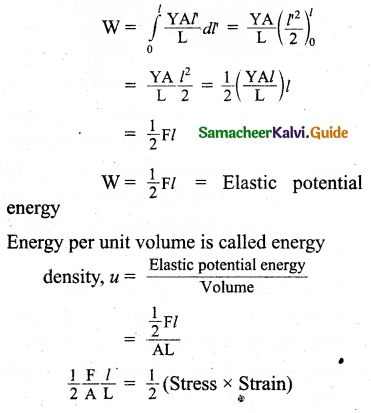Question 4.
Derive an equation for the total pressure at a depth ‘h’ below the liquid surface.
Let us consider a water sample of a cross-sectional area in the form of a cylinder. Let h1 and h2 be the depths from the air-water interface to level 1 and level 2 of the cylinder, respectively as shown in Figure (a). Let F1 be the force acting downwards on level 1 and F2 be the force acting upwards on level 2, such that, F1 = P1 A and F2 = P2 A Let the mass of the sample to be m and under equilibrium condition, the total upward force (F2) is balanced by the total downward force (F1 + mg), otherwise, the gravitational force will act downward which is being exactly balanced by the difference between the force F2 – F1
F2 – F1 = mg = FG … (1)
Where m is the mass of the water available in the sample element. Let p be the density of the water then, the mass of water available in the sample element is
m = ρV = ρA(h2 – h1])
V= A (h2 – h1)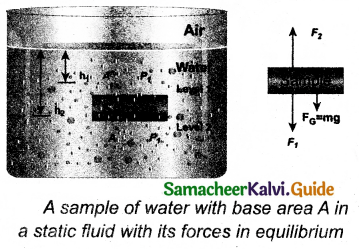A sample of water with base area A in a static fluid with its forces in equilibrium
Hence, gravitational force,
FG = ρA(h2 – h1)g
On substituting the value of W in equation (1)
F2 = F1 + mg
⇒ P2A = P1A + ρA(h2 – h1)g
Cancelling out A on both sides,
P2 = P1 + ρ (h2 – h1)g … (2)If we choose level 1 at the surface of the liquid (i.e., air-water interface) and level 2 at a depth ‘h ’ below the surface (as shown in Figure), then the value of h1 becomes zero (h1 = 0) when P1 assumes the value of atmospheric pressure (say Pa). In addition, the pressure (P2) at a depth becomes P. Substituting these values in the equation,
p2 = p1 + ρ(h2 – h1)g
we get
p = pa + ρgh
This means, the pressure at a depth h is greater than the pressure on the surface of the liquid, where Pa is the atmospheric pressure = 1.013 x 105 Pa. If the atmospheric pressure is neglected then
p = ρ gh
For a given liquid, p is fixed and g is also constant, then the pressure due to the fluid column is directly proportional to vertical distance or height of the fluid column.Question 5.
State and prove Pascal’s law in fluids.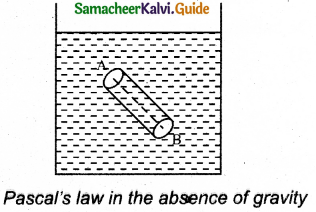Pascal’s law states that if the effect of gravity can be neglected then the pressure in a fluid in equilibrium is the same everywhere. Let us consider any two points A and B inside the fluid imagined. A cylinder is such that points A and B lie at the centre of the circular surface at the top and bottom of the cylinder.

Let the fluid inside this cylinder be in equilibrium under the action of forces from outside the fluid. The forces acting on the circular, top, and bottom surfaces are perpendicular to the forces acting on the cylindrical surface. Therefore the forces acting on the faces at A and B are equal and opposite and hence add to zero.

As the areas of these two faces are equal, the pressure at A = pressure at B. This is the proof of Pascal’s law when the effect of gravity is not taken into account.

Question 6.
State and prove Archimedes principle.Archimedes principle states that when a body is partially or wholly immersed in a fluid, it experiences an upward thrust equal to the weight of the fluid displaced by it, and its upthrust acts through the centre of gravity of the liquid displaced.
Proof:
Consider a body of height h lying inside a liquid of density p, at a depth x below the free surface of the liquid. The area of a cross-section of the body is a. The forces on the sides of the body cancel out.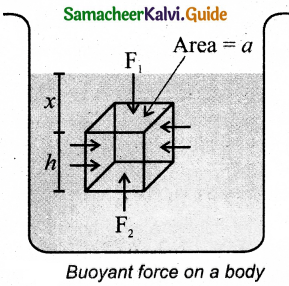Pressure at the upper face of the body,
P1 = xρg
Pressure at the lower face of the body,
P2 = (x + h)pρg
Thrust acting on the upper face of the body is
F1 = P1a = xρga
acting vertically downwards.
Thrust acting on the lower face of the body is
F2 = P2a = (x+h)ρga
acting vertically upwards.
The resultant force (F2 – F1) is acting on the body in the upward direction and is called Upthrust (U).
∴ U = F2 – F1 = (x + h)ρg – xρga = ahρg
But, ah = V, the volume of the body = volume of liquid displaced.
U = Vρg = Mg
[∴ M = Vρ = mass of liquid displaced] i.e., upthrust or buoyant force.
= Weight of liquid displaced.
This proves the Archimedes principle.

Question 7.
Derive the expression for the terminal velocity of a sphere moving in a high viscous fluid using stokes force.
Let us consider a sphere of radius r which falls freely through a highly viscous liquid of coefficient of viscosity η. Let the density of the material of the sphere be p and the density of the fluid be c.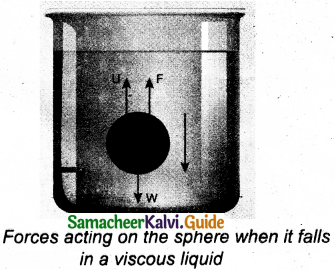Gravitational force acting on the sphere,
FG = mg = $$\frac { 4 }{ 3 }$$πr³pg (downward force)
Upthrust, U= $$\frac { 4 }{ 3 }$$πr³σg (upward force)
viscous force F = 6πηrvt
At terminal velocity vt.
downward force = upward force.Question 8.
Derive Poiseuille’s formula for the volume of a liquid flowing per second through a pipe under streamlined flow.
Consider a liquid flowing steadily through a horizontal capillary tube.
Let v = ($$\frac { V }{ t }$$) be the volume of the liquid flowing out per second through a capillary tube.
It depends on
(i) coefficient of viscosity (η) of the liquid
(ii) radius of the tube (r).
(iii) the pressure gradient (yj Then,So, equating the powers of M, L, and T on both sides, we get
a + c = 0, – a + b – 2c = 3, and – a – 2c = 1 On solving three equations, we get
a = – 1, b = 4, and c = 1
Therefore, equation (1) becomes,
v = kη-1r($$\frac { p }{ l }$$)1
Experimentally, the value of k is shown to be $$\frac { π }{ 8 }$$, we have
V = $$\frac{\pi r^{4} \mathrm{P}}{8 \eta l}$$
The above equation is known as Poiseuille’s equation for the flow of liquid through a narrow tube or a capillary tube.

Question 9.
Obtain an expression for the excess of pressure inside a
(i) liquid drop
(ii) liquid bubble
(iii) air bubble.
(i) Liquid drop: Let us consider a liquid drop of radius R and the surface tension of the liquid is T.The various forces acting on the liquid drop are,
(a) Force due to surface tension FT= 2πRT towards the right
(b) Force due to outside pressure, F$$\mathrm{F}_{\mathrm{P}_{1}}$$ towards right
(c) Force due to inside pressure,
$$\mathrm{F}_{\mathrm{P}_{2}}$$ = P2 πR² towards left
As the drop is in equilibrium,
$$\mathrm{F}_{\mathrm{P}_{2}}$$ = FT + $$\mathrm{F}_{\mathrm{P}_{1}}$$
P22πR² = 2πRT + P1πR²
⇒ (P2 – P1)πR² = 2πRT
Excess pressure is ∆P = P2 – P1 = $$\frac { 2T }{ R }$$

(ii) Liquid bubble: A soap bubble of radius R and the surface tension of the soap bubble be T is as shown in Figure. A soap bubble has two liquid surfaces in contact with air, one inside the bubble and the other outside the bubble. Hence, the force on the soap bubble due to surface tension is 2 x 2πRT. The various forces acting on the soap bubble are,(a) Force due to surface tension
FT = 4πRT towards right

(b) Force due to outside pressure,
FP = P1πR² towards right

(c) Force due to inside pressure,
$$\mathrm{F}_{\mathrm{P}_{2}}$$ = P2πR² towards left
As the bubble is in equilibrium,
$$\mathrm{F}_{\mathrm{P}_{2}}$$ = FT + $$\mathrm{F}_{\mathrm{P}_{1}}$$
P2πR² = 4πRT + P1πR²
⇒ (P2 – P1)πR² = 4πRT
Excess pressure is ∆P = P2 – P1 = $$\frac { 4T }{ R }$$

(iii) Air bubble: Let us consider an air bubble of radius R inside a liquid having surface tension T as shown in Figure. Let P1 and P2 be the pressures outside and inside the air bubble, respectively. Now, the excess pressure inside the air bubble is ∆P = P1 – P2.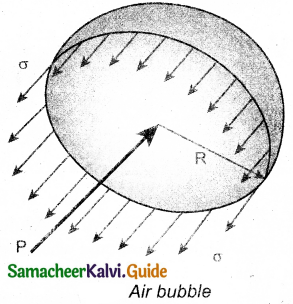In order to find the excess pressure inside the air bubble, let us consider the forces acting on the air bubble. For the hemispherical portion of the bubble, considering the forces acting on it, we get,
(a) The force due to surface tension acting towards right around the rim of length 2πR is FT = 2πRT

(b) The force due to outside pressure P1 is to the right acting across a cross-sectional area of πR² is $$\mathrm{F}_{\mathrm{P}_{1}}$$ = P1πR²

(c) The force due to pressure P2 inside the bubble, acting to the left is $$\mathrm{F}_{\mathrm{P}_{2}}$$ = P2πR²
As the air bubble is in equilibrium under the action of these forces, $$\mathrm{F}_{\mathrm{P}_{2}}$$ = FT + $$\mathrm{F}_{\mathrm{P}_{1}}$$.Question 10.
What is capillarity? Obtain an expression for the surface tension of a liquid by the capillary rise method.
The rise or fall of a liquid in a narrow tube is called capillarity. Let us consider a capillary tube which is held vertically in a beaker containing water; the water rises in the capillary’ tube to a height h due to surface tension.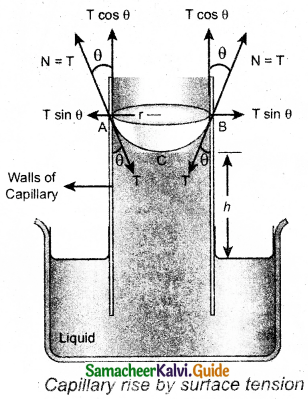The surface tension force FT, acts along the tangent at the point of contact downwards and its reaction force upwards. Surface tension T is resolved into two components.
(i) Horizontal component T sinθ and
(ii) Vertical component T cosθ acting upwards, all along the whole circumference of the meniscus.
Total upward force = (T cosθ) (2πr)
= 2πrT. cosθ
Where θ is the angle of contact, r is the radius of the tube. Let ρ be the density of water and h be the height to which the liquid rises inside the tube. Then,The upward force supports the weight of the liquid column above the free surface, therefore,If the capillary is a very fine tube of the radius (i.e., the radius is very small) then $$\frac { r }{ 3 }$$ can be neglected when it is compared to the height h. Therefore,
T = $$\frac{r \rho g h}{2 \cos \theta}$$

Question 11.
Obtain an equation of continuity for a flow of fluid on the basis of conservation of mass.
Let us consider a pipe AB of varying cross-sectional areas a1 and a2 such that a1 > a2. A non – viscous and incompressible liquid flows steadily through the pipe, with velocities v1 and v2 in areas a1 and a2, respectively as shown in Figure.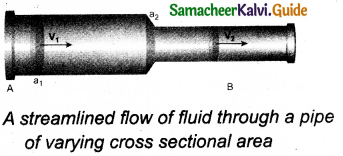Let m1 be the mass of fluid flowing through section A in time ∆t, m1 = (a1v1 ∆t)ρ
Let m2 be the mass of fluid flowing through section B in time ∆t, m2 = (a2v2 ∆t)ρ
For an incompressible liquid, mass is conserved m1 = m2
a1v1 ∆tρ = a2v2∆tρ
a1v1 = a2v2 ⇒ av = constant
Which is called the equation of continuity. It is based on the conservation of mass in the flow of fluids. In general, av – constant.

Question 12.
State and prove Bernoulli’s theorem for a flow of incompressible, non-viscous, and streamlined flow of fluid.
Statement:
According to Bernoulli’s theorem, the sum of pressure energy, kinetic energy, and potential energy per unit mass of an incompressible, non-viscous fluid in a streamlined flow remains a constant.Proof:
Let us consider a flow of liquid through a pipe AB as shown in Figure. Let V be the volume of the liquid when it enters A in a time t which is equal to the volume of the liquid leaving B in the same time. Let aA, vA, and PA. be the area of cross-section of the tube, velocity of the liquid, and pressure exerted by the liquid at A respectively.
Let the force exerted by the liquid at A is
FA = VA
Distance travelled by the liquid in time t is
d = vA t
Therefore, the work done is
W = FAd = PA aA vA t
But aA vA t = aA d = V, the volume of the liquid entering at A.
Thus, the work done is the pressure energy (at A),
W = FA d = PA V
Pressure energy per unit mass at pressure energy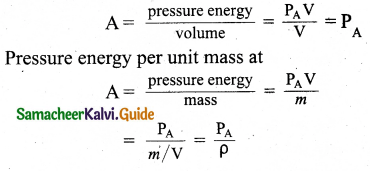Since m is the mass of the liquid entering at A in a given time, therefore, pressure energy of the liquid at A is
EPA = PAV = PAV x $$\frac { m }{ m }$$ = m $$\frac{P_{A}}{\rho}$$
Potential energy of the liquid at A,
PA = mg hA,
Due to the flow of liquid, the kinetic energy of the liquid at A,
KEA = $$\frac { 1 }{ 2 }$$mv²A
Therefore, the total energy due to the flow of liquid at A, EA = EPA + KEA + PEA
EA = $$m \frac{\mathrm{P}_{\mathrm{A}}}{\rho}+\frac{1}{2} m v_{\mathrm{A}}^{2}+m g h_{\mathrm{A}}$$
Similarly, let aB, vB, and PB be the area of a cross section of the tube, the velocity of the liquid, and pressure exerted by the liquid at B. Calculating the total energy at EB, we get
EB = $$m \frac{\mathrm{P}_{\mathrm{B}}}{\rho}+\frac{1}{2} m v_{\mathrm{B}}^{2}+m g h_{\mathrm{B}}$$
From the law of conservation of energy,Thus, the above equation can be written as
$$\frac { p }{ ρg }$$ + $$\frac { 1 }{ 2 }$$$$\frac{v^{2}}{g}$$ + h = constant

Question 13.
Describe the construction and working of venturimeter and obtain an equation for the volume of liquid flowing per second through a wider entry of the tube.
Construction: It consists of two wider tubes A and A’ (with cross-sectional area A) connected by a narrow tube B (with the cross-sectional area a). A manometer in the form of a U-tube is also attached between the wide and narrow tubes as shown in Figure. The manometer contains a liquid of density ‘ρm‘.Theory:
Let P1 be the pressure of the fluid at the wider region of tube A. Let us assume that the fluid of density ‘ρ’ flows from the pipe with speed ‘v1’ and into the narrow region, its speed increases to ‘v1‘.

According to Bernoulli’s equation, this increase in speed is accompanied by a decrease in the fluid pressure P2 at the narrow region of the tube B. Hence, the pressure difference between tubes A and B is noted by measuring the height difference (∆P = P1 – P2) between the surfaces of the manometer liquid.
From the equation of continuity, we can say that Av1 = av2 which means that
v2 = $$\frac { A }{ a }$$v1
Using Bernoulli’s equation,IV. Numerical Problems:

Question 1.
A capillary of diameter d mm is dipped in water such that the water rises to a height of 30 mm. If the radius of the capillary is made ($$\frac { 2 }{ 3 }$$)of its previous value, then compute the height up to which water will rise in the new capillary?
Let diameter of capillary tube = d mm
Let the radius of capillary tube r1 = r mm
Capillary rise h1 = 30 mm
Let the radius of another capillary tube r2 = $$\frac { 2 }{ 3 }$$ r
Let the capillary rise of another capillary tube be h2
We know that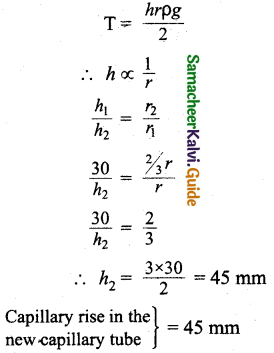Question 2.
A cylinder of length 1.5 m and diameter 4 cm is fixed at one end. A tangential force of 4 x 105 N is applied at the other end. If the rigidity modulus of the cylinder is 6 x 105 Nm-2 then, calculate the twist produced in the cylinder.
Length of a cylinder l = 1.5 m
Diameter of a cylinder d = 4 x 10-2 m
Tangential force Ft = 4 x 105 N
Rigidity modulus ηR = 6 x 1010 Nm-2
Twist produced θ = ?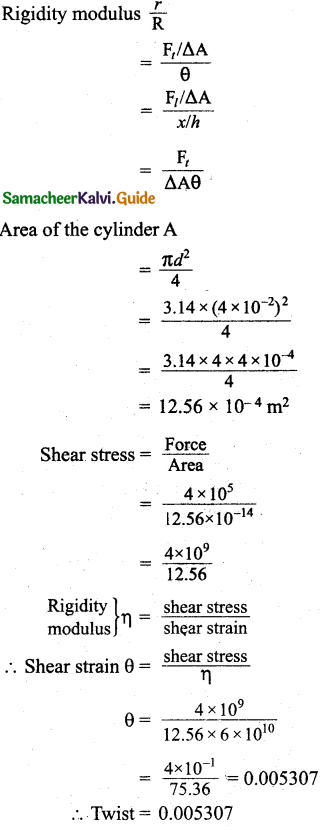Question 3.
A spherical soap bubble A of radius 2 cm is formed inside another bubble B of radius 4 cm. Show that the radius of a single soap bubble which maintains the same pressure difference as inside the smaller and outside the larger soap bubble is lesser than the radius of both soap bubbles A and B.
Excess of pressure inside the liquid due to surface tension,
∆P = $$\frac { 2T }{ R }$$
Where T – surface tension
In the case of soap bubbles, the excess pressure inside the soap bubble,
∆Pb = $$\frac { 4T }{ R }$$
Excess of pressure of air inside the bigger bubble
∆Pbigger = $$\frac { 4T }{ 4 }$$ = T
Excess of pressure of air inside the smaller bubble
∆Psmaller = $$\frac { 4T }{ 2 }$$ = 2T
Air pressure different between the smaller bubble and the atmosphere will be equal to the sum of excess pressure inside the bigger and smaller bubbles.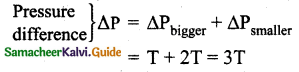Excess pressure inside a single soap bubble
= $$\frac { 4T }{ R }$$ = 4T = T
∴ Pressure different of single soap bubble less than the radius of both T < 3T.Question 4.
A block of Ag of mass x kg hanging from a string is immersed in a liquid of relative density 0.72. If the relative density of Ag is 10 and tension in the string is 37.12 N then compute the mass of the Ag block.
Let the mass of Ag block be x kg,
Tension in the string T = 37.12 N.
Relative density of liquid Rliq = 0.72
Relative density of silver RAg = 10
According to the principle of flotation
Vpg = mg
∴ Vp=m … (1)
Weight of Ag block = mg = Vρg = RAgVg
Weight of Ag block W = 10 Vρg [ ∵ m = Vρ]
Force of buoyancy FB = Rliq (Vρ)g = 0.72 Vρg
Apparent weight Wapp = 10 Vρg – 0.72 Vρg
= 9.78 Vρg
= 9.78 mg
Tension in the string = Apparent weight
37.12= 9.78 mg
Mass m = $$\frac { 37.12 }{ 9.28 }$$ = 4 kg

Question 5.
The reading of the pressure meter attached with a closed pipe is 5 x 105 Nm-2. On opening the valve of the pipe, the reading of the pressure meter is 4.5 x 105 Nm-2. Calculate the speed of the water flowing in the pipe.
Initial pressure P2 = 5 x 105 Nm-2
Final pressure P1 = 4. 5 x 105 Nm-2
Initial velocity V1 = 0
Final velocity v2 = v2
Density of water ρ = 10³ kg/m³
Using Bernoulli’s theorem, we can write,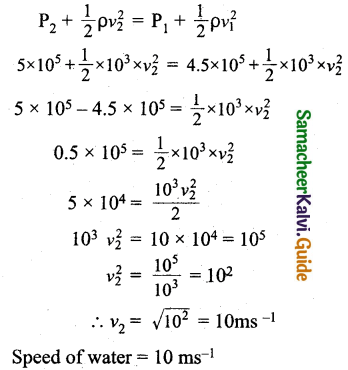V. Conceptual Questions:

Question 1.
Why coffee runs up into a sugar lump (a small cube of sugar) when one corner of the sugar lump is held in the liquid?
Dip the comer of a sugar cube in coffee, and get the whole cube coffee-flavoured due to “capillary action”.

Question 2.
Why two holes are made to empty an oil tin?
When oil comes out of one hole with high velocity, the pressure in the tin decreases. To have a continuous flow of oil proper pressure is to be maintained inside the tin. To achieve this, atmospheric air has to be entered inside the tin. For this purpose, only another hole is made out. Hence two holes are made to empty an oil tin.

Question 3.
We can cut vegetables easily with a sharp knife as compared to a blunt knife. Why?